I started taking “Fundamentals of Music Theory “ on Coursera. Summary of week 1. So far there are a lot of stuff “just by definition”, so I honestly felt a need to wrote a summary to structure everything in my head (since I already forgot quite a lot).

We can compare sounds and we call them low and high. Some sounds we can’t sing. For the ones we can, we say they have pitch.

We denote notes using letters A, B, C, D, E, F, G. This is our music alphabet.

### Notation

One can write relative changes in pitch, but this is still ambiguous for a reader, if they don’t know any absolute value. Thus, monks struggled with writing down and transmitting melodies. As a result, stave notation was born.

Stave is based on 5 horizontal lines. A symbol on the left is called G-clef or Treble-clef. It circles itself on line representing note G. Each line and space between lines represent a note (a white key on piano keyboard).Mnemonics:

• spaces between lines starting from bottom - F A C E
• lines starting from bottom - Every Good Boy Deserves Fruit

One can add additional lines when leaving stave’s range. E.g. middle C is the first line under the stave.

However, we have 12 pitches on guitar (i.e. also black keys on piano keyboard) in one octave.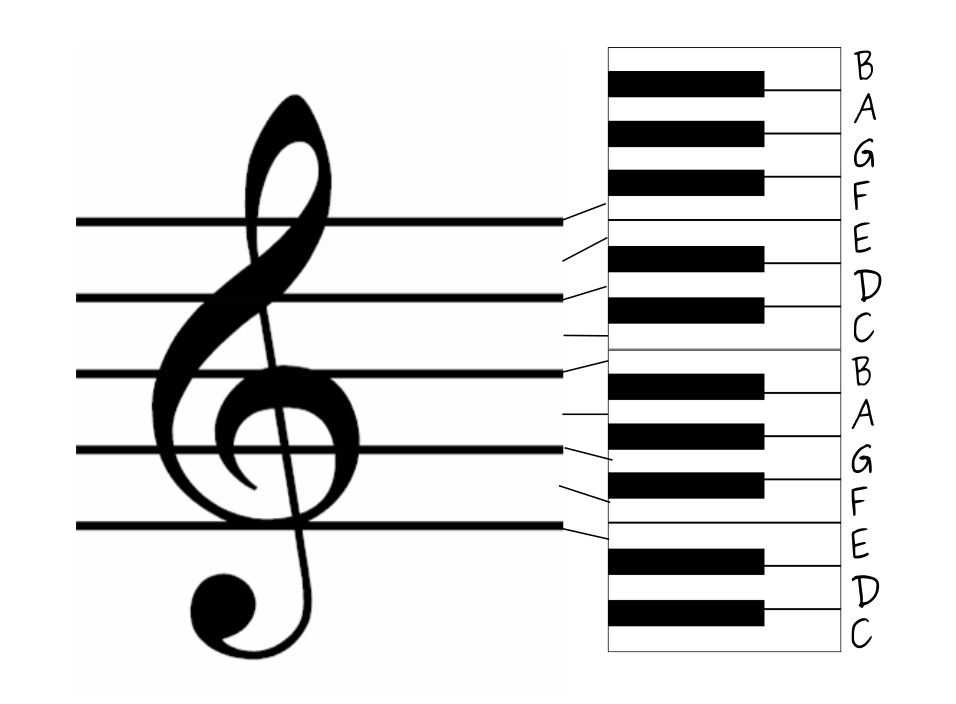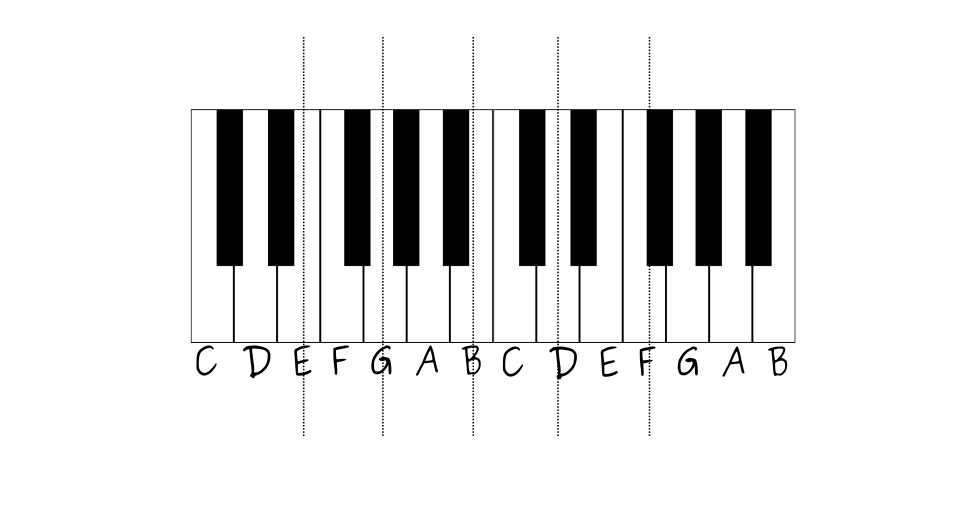## Intervals

Octave - interval between (and including) two notes, one having twice or half the vibration frequency of the other.

Semitone - distance between two adjacent keys on piano.

Tone = 2 semitones.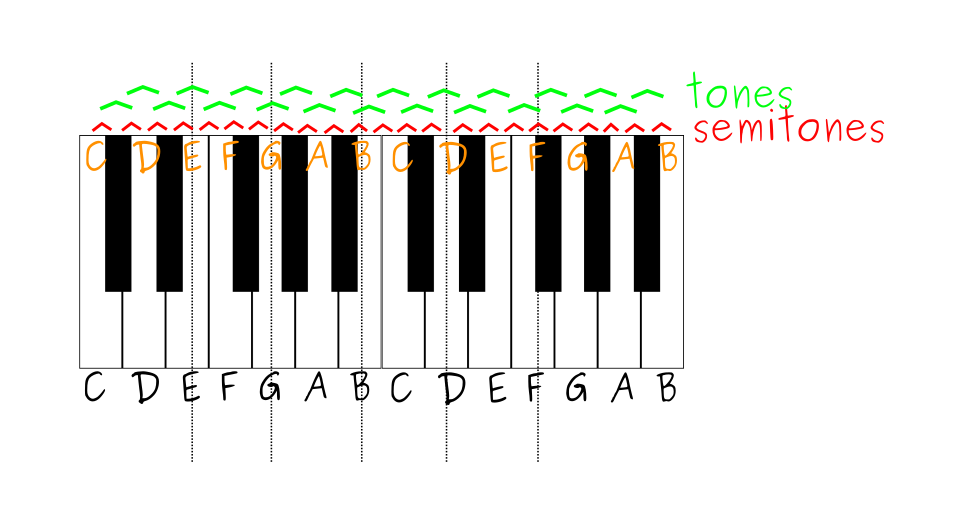Let’s call one line or space in stave - a unit. We say that interval between two notes is “a second” if there are no units between them on the stave (i.e. this is a line and its adjacent space).

When there is one unit - “a third” ( i.e. two lines with one space in between or two spaces with one line).

Here comes a bit confusing part. F to G is a second. C to B is a second. There is a black key between F and G, but not between C & B. Nevertheless both are called - “a second”.

## Scales

Scale - a pathway through octave.

Example - C Major (we just go through notes one by one)

C <T> D <T> E <S> F <T> G <T> A <T>> B <S> C

where <T> is a tone and <S> is a semitone.

C is called a tonic.

Sequence TTSTTTS defines “quality of scale”. This one belongs to Diatonic Scale (because it has 5 tones and 2 semitones).

There are different approaches in the world, this one is so called common practice, i.e. Western Europe 1600-1900.

If we take A as a tonic and again go through notes one by one, we get natural minor scale

A <T> B <S> C <T> D <T> E <S> F <T> G <T> A

i.e. the sequence of T’s and S’s is different from major This is still Diatonic Scale (still 5 T and 2 S). The sequence itself is called Aeolian mode.

When starting from D, we get Dorian mode

D <T> E <S> F <T> G <T> A <T> B <S> C <T> D

E -> Phrygian

E <S> F <T> G <T> A <T> B <S> C <T> D <T> E

Basically we just traverse the stave starting from a note and write down all the notes we meet on our way.

F -> Lydian.

G -> Mixolydian (also 5th degree of C Major, interval between C and G is a fifth).

B -> Locrian mode.

## Chords

Let’s take a piece of natural minor scale

A B C D E

and count semitones

A <T - 1 2> B <S - 3> C <T - 4 5> D <T - 6 7> E

We can do the same for C Major

C <T - 1 2> D <T 3 4> E <S 5> F <T 6 7> G

7 semitones = perfect fifth

A -> C = a minor third (3 semitones)

C -> E = a major third (4 semitones)

C Major chord is C E G (C major triad).

Abbreviations:

• C Major = C, Cmaj, CM
• A Minor = Amin, Am, A-

One can construct a major triad by taking major third and perfect fifth (i.e. a note 4 semitones away and a note 7 semitones away).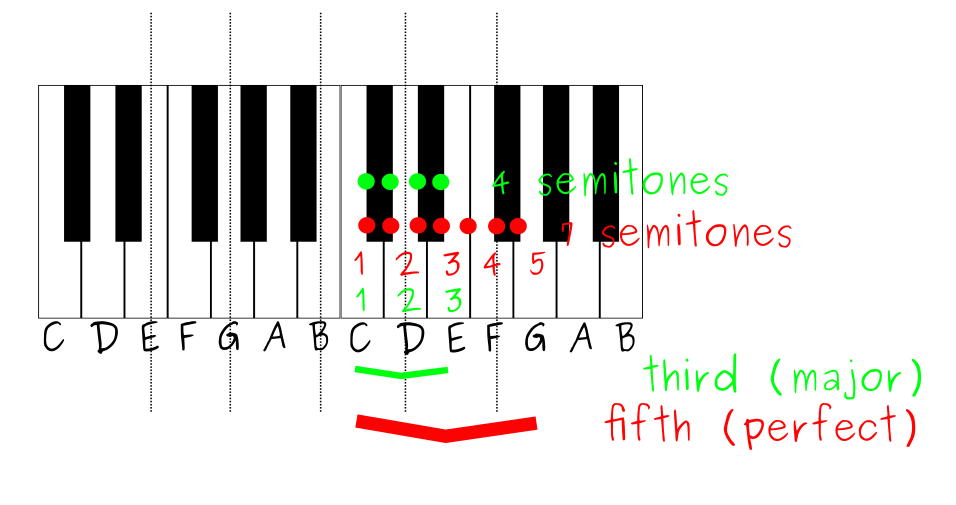For a minor triad - minor third (3 semitones) + perfect fifth (7 semitones).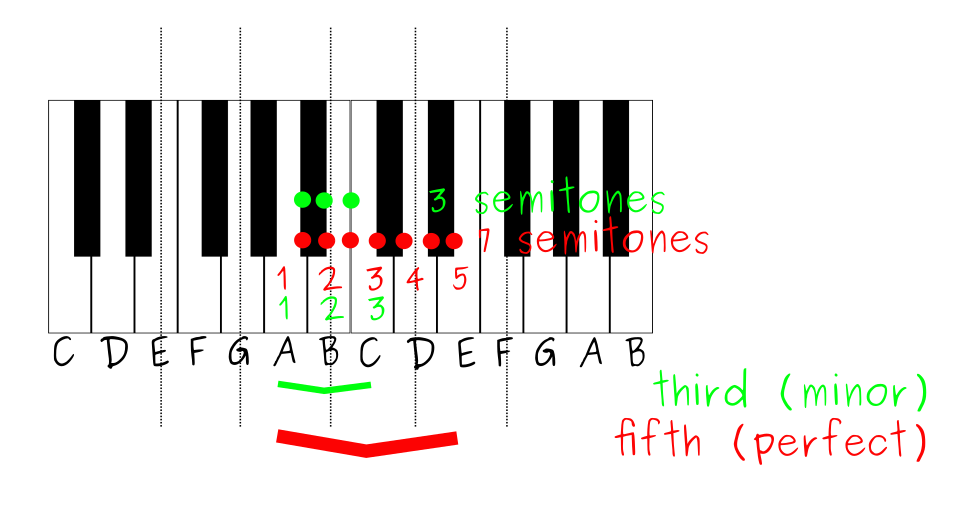There is also Diminished Triad (B0 Bdim) = Minor third (3 semitones) + Diminished Fifth (6 semitones).

Note that “third” and “fifth” are taken from the stave, but whether it is “perfect”, “minor”, “diminished” is based on the underlying notes. I.e. we take C, count one stave unit, get E, count another stave unit - get G. Now we can count semitones and identify whether this is maj, min or dim.

If we repeat taking third and fifth from every note, we get:

Cmaj Dmin Emin Fmaj Gmaj Amin Bdim

### Three Chord Trick

C, F and G work well together.

• C - tonic triad (I)
• F - subdominant (IV) - the tonic is domimant of the subdomimant. It is as far below the tonic as domimant is above.
• G - 5th degree (C -> G is a fifth) - dominant (V)

You can harmonize notes with chords which have it.